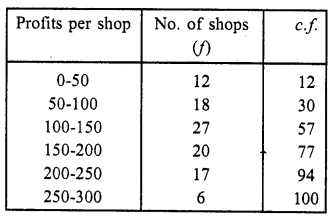• +91 9971497814
• info@interviewmaterial.com

# RD Chapter 7- Statistics Ex-7.6 Interview Questions Answers

### Related Subjects

Question 1 : Draw an ogive by less than method for the following data :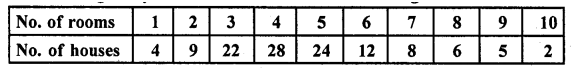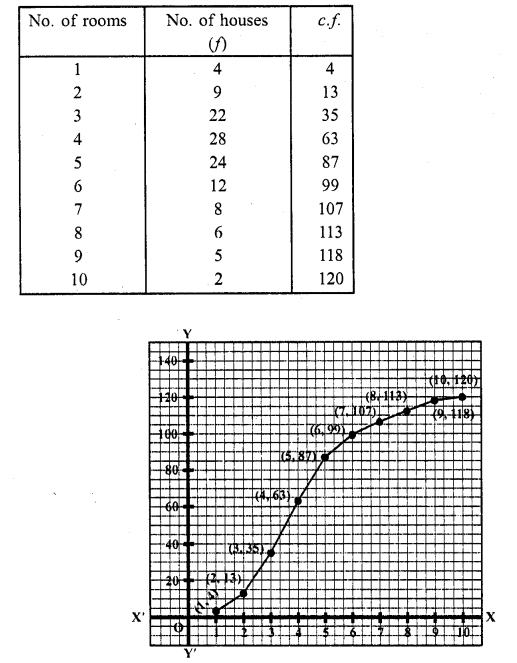Take numbers of rooms along the x-axis and c.f along the y-axis
Plot the points (1, 4), (2, 13), (3, 35), (4, 63), (5, 87), (6, 99), (7, 107), (8, 113), (9, 118) and (10, 120) on the graph and join them and with free hand to get an ogive as shown. This is the less than ogive.

Question 2 :
The marks scored by 750 students in an examination are given in the form of a frequency distribution table: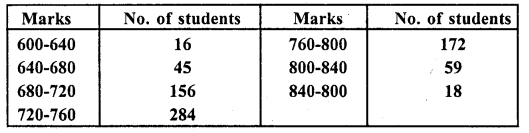Prepare a cumulative frequency table by less than method and draw on ogive.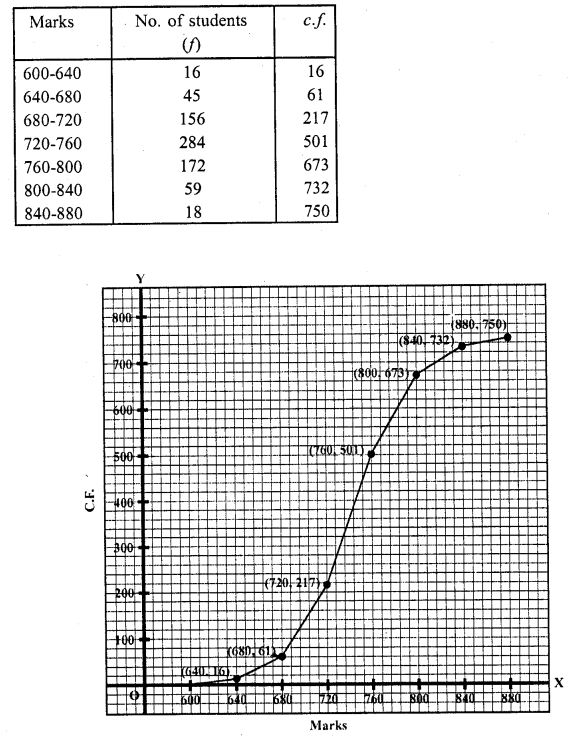Take marks along ,t-axis and no. of students (c.f) along 3 -axis. Now plot the points (640, 16), (680, 61), (720, 217), (760, 501), (800, 673), (840, 732) and (880, 750) on the graph and join them with free hand. This is the less than ogive.

Question 3 : Draw an ogive to represent the following frequency distribution: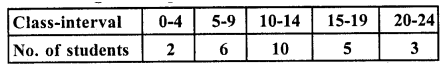Representing the classes in exclusive form :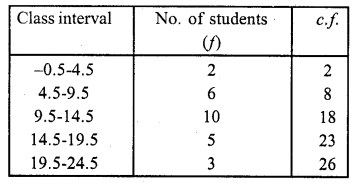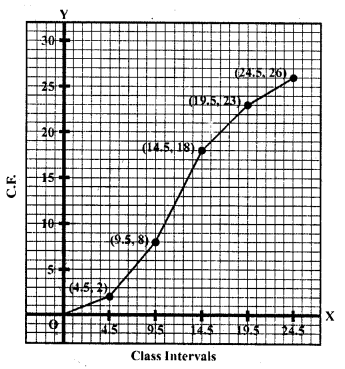Represent class intervals along x-axis and c.f. along y-axis. Now plot the points (4.5, 2), (9.5, 8), (14.5, 18), (19.5, 23) and (24.5, 26) on the graph and join then in free hand to get an ogive as shown.

Question 4 :
The monthly profits (in Rs.) of 100 shops are distributed as follows: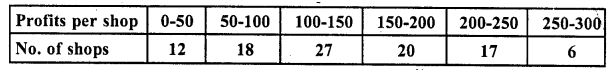Draw the frequency polygon for it.